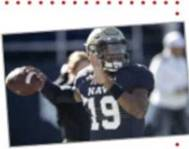Chapter 12.3, Problem 32E

Chapter
Section
Textbook Problem

# Projectile Motion In Exercises 27-40, use the model for projectile motion, assuming there is no air resistance and g = 32 feet per second per second.FootballThe quarterback of a football team releases a pass at a height of 7 feet above the playing field, and the football is caught by a receiver 30 yards directly downfield at a height of 4 feet The pass is released at an angle of 35 ∘ with the horizontal.(a) Find the speed of the football when it is released.(b) Find the maximum height of the football.(c) Find the time the receiver has to reach the proper position after the quarterback releases the football.

(34) (a)

To determine
The released speed of the football when a pass at a height of 7 feet above the playing field, at an angle of 35° with the horizontal.

Explanation

Given:

The provided data are h=7 feet, x=30 yeards, θ=35.

Whereas, h is height above the playing field, x is height of receiver and θ is an angle.

Explanation:

Let the velocity be v ft/s

we know that the equation of a projectile motion is y=gsec2θ2v02x2+tanθx+hhere the given values areθ=35°,x=30yards=90feet,h=7feet,y<

(b)

To determine
The Maximum height of the football when a pass at a height of 7 feet above the playing field, at an angle of 35° with the horizontal.

(c)

To determine
The time the receiver has to reach the proper position after the quarterback releases the football.

### Still sussing out bartleby?

Check out a sample textbook solution.

See a sample solution

#### The Solution to Your Study Problems

Bartleby provides explanations to thousands of textbook problems written by our experts, many with advanced degrees!

Get Started

#### Find more solutions based on key concepts